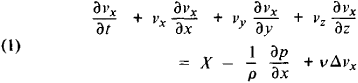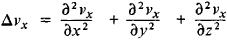Navier-Stokes Equations

Also found in: Wikipedia.

Navier-Stokes equations

[nä′vyā ′stōks i‚kwā·zhənz]
(fluid mechanics)
The equations of motion for a viscous fluid which may be written d V/ dt = -(1/ρ)∇ p + F + ν∇2V + (⅓)ν∇(∇·V), where p is the pressure, ρ the density, F the total external force per unit mass, V the fluid velocity, and ν the kinematic viscosity; for an incompressible fluid, the term in ∇·V (divergence) vanishes, and the effects of viscosity then play a role analogous to that of temperature in thermal conduction and to that of density in simple diffusion.

Navier-Stokes Equations

the differential equations that describe the motion of a viscous fluid. These equations are named after L. Navier and G. Stokes. For an incompressible (density ρ = constant) and unheated (temperature T = constant) fluid, the Navier-Stokes equations projected on the axes of a rectangular Cartesian coordinate system (a system of three equations) have the formHere t is the time; x, y, and z are the coordinates of a particle of fluid; vx, vy, and vz are the projections of the velocity of the particle; X, Y, and Z are the projections of the body force; ρ is the pressure; ν = μ/ρ is the kinematic viscosity coefficient (where μ is the dynamic viscosity coefficient), andTwo other equations are obtained by replacing x with y, y with z, and z with x.

The Navier-Stokes equations are used to determine vx, vy vz., and ρ as functions of x, y, z, and t. In order to close the system, we add to equations (1) a continuity equation, which for an incompressible fluid has the formIn order to integrate equations (1) and (2), we must be given the initial conditions (if the motion is not steady state) and the boundary conditions, which for a viscous fluid are the conditions of adhesion to rigid walls. In the general case of the motion of a compressible and heated fluid, the Navier-Stokes equations also take into account the variability of ρ and the temperature dependence of μ, changing the form of the equations. In this case, the equation of energy balance and the Clapeyron equation are also used.

The Navier-Stokes equations are used in the study of the motions of real liquids and gases; in most such specific problems, only various approximate solutions are sought.

S. M. TARG

References in periodicals archive ?
Most fluids on Earth are nonconductive and can be described by the Navier-Stokes equations, say Beresnyak and Lazarian, so most turbulence studies are on such fluids.
LBM is a computational fluid dynamics mesoscale system that instead of solving the Navier-Stokes equations, the discrete Boltzmann equation is used to simulate the flow of a Newtonian fluid by modelling streaming and collision of particles within the flow.
Traditional CFD can model essentially all the relevant airflow physics by solving a simplified form of the full Navier-Stokes equations (Kang et al., 2000; Karki et al., 2003; VanGilder & Schmidt, 2005; Pardey et al., 2015).
First, background on the use of full Navier-Stokes equations to model the hydrology and resulting WFT on road surfaces is described, followed by the definitions of the PE and the PM.
, where the convergence of (local) smooth solution of the NSPK system with viscosity term [mu] [DELTA] [u.sup.[lambda]] + v[nabla] [divu.sup.[lambda]] to the smooth solution of the incompressible Navier-Stokes equations is obtained by using the convergence-stability principle.
Only a few investigations used the calculation of the Navier-Stokes equations with finite volume method  or infinite element method [12, 14].
The thermoacoustic wave propagation is modelled by the compressible Navier-Stokes equations. These equations can be expressed in a vector conservation form as follows:
The aim of this study is to investigate the hemodynamic parameter, the pressure distribution, and wall shear stress in a stenosed artery using Reynolds-averaged Navier-Stokes equations for turbulence fluid modelling and Newtonian equations for the translation and rotational motion of the bioparticles.
This work concerns the existence and uniqueness of global strong solutions and sharp decay estimates of solutions to initial-boundary value problems for the 4D Navier-Stokes equations:
And the Stokes operator [A.sub.p] is of BIP type with the power angle [mathematical expression not reproducible] (see, we can make [L.sup.p] - [L.sup.q] estimates for the parabolic evolution equations (see [12, 13]) and the Navier-Stokes equations (see [14,15]).
Generalized Navier-Stokes Equation. The governing equations of mass and momentum for the generalized Navier-Stokes equations can be given by
Different upwind schemes have been developed for the multidimensional Navier-Stokes equations. Razavi et al.

Site: Follow: Share:
Open / Close